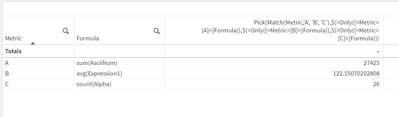# App Development

Announcements
Customer & Partners, DEC. 9, 11 AM ET: Qlik Product & Strategy Roadmap Session: Data Analytics REGISTER NOW
cancel
Showing results for
Did you mean:Contributor II

## How to calculate formulas stored in a column?

Imagine you have a data island table with two columns, e.g. "Metric" and "Function". The function column contains a syntactically correct formula per metric which calculates its result over the remaining tables of the data model. Then create a table object in the UI and have Metric as dimension and the expression "\$(=[Function])" as measure column. The output is an empty measure column unless I select one of the metrics in the dimension columns, then Qlik Sense Enterprise SaaS shows the correct result for the chosen metric. How do I need to formulate the expression so that it calculates the results for all rows right away? An empty sample QVF using the data model generated by hitting CTRL-0-0 in the load editor is attached

Thanks,

Stefan
Labels (2)

• ### SaaS

1 Solution

Accepted SolutionsMVP

This doesn't work because \$ expansion happens outside the chart. That means when you have multiple measures in scope (not selected and reduced to one - which you see works), the \$ expansion can't produce multiple row-wise expressions (which is desired). As an alternative, you could try with pick(match(... Something like:

Pick(Match(Metric,'A', 'B', 'C'),\$(=Only({<Metric={A}>}Formula)),\$(=Only({<Metric={B}>}Formula)),\$(=Only({<Metric={C}>}Formula)))And to make it dynamic, try using Concat() to produce the expression.

2 RepliesMVP

This doesn't work because \$ expansion happens outside the chart. That means when you have multiple measures in scope (not selected and reduced to one - which you see works), the \$ expansion can't produce multiple row-wise expressions (which is desired). As an alternative, you could try with pick(match(... Something like:

Pick(Match(Metric,'A', 'B', 'C'),\$(=Only({<Metric={A}>}Formula)),\$(=Only({<Metric={B}>}Formula)),\$(=Only({<Metric={C}>}Formula)))And to make it dynamic, try using Concat() to produce the expression.Contributor II
Author

Thanks  @tresesco that was exactly the problem, your solution works perfectly and dynamically using concat().  Do you happen to know the max length of the concatenated string?

Thanks,

StefanTags
Community Browser